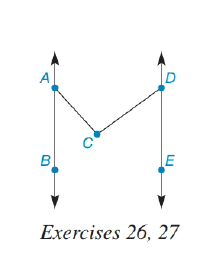Chapter 2.1, Problem 24E### Elementary Geometry for College St...

6th Edition
Daniel C. Alexander + 1 other
ISBN: 9781285195698

#### Solutions

Chapter
Section### Elementary Geometry for College St...

6th Edition
Daniel C. Alexander + 1 other
ISBN: 9781285195698
Textbook Problem
1 views

# Given: A B ↔ ∥ D E ↔ m ∠ B A C = 42 ° m ∠ E D C = 54 ° Find: m ∠ A C D(HINT: There is a line through C parallel to both A B ↔ and D E ↔ .)

To determine

To find:

The value of mACD.

Explanation

Given:

The given figure is,

Figure (1)

And,

ABDE, BAC=42° and EDC=54°.

Concept:

If two parallel lines are cut by a transversal then the angles of the alternate sides of transverse lines are equal.

Construction:

Draw a straight line passing through point C in such a way that it is parallel to lines AB and DE and name it as FG.

Figure (2)

Calculation:

Consider the figure (2),

mACF+mDCF=mACD ……(1)

Alternate angles are congruent

### Still sussing out bartleby?

Check out a sample textbook solution.

See a sample solution

#### The Solution to Your Study Problems

Bartleby provides explanations to thousands of textbook problems written by our experts, many with advanced degrees!

Get Started